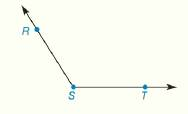Chapter 1.CR, Problem 13CR### Elementary Geometry for College St...

6th Edition
Daniel C. Alexander + 1 other
ISBN: 9781285195698

#### Solutions

Chapter
Section### Elementary Geometry for College St...

6th Edition
Daniel C. Alexander + 1 other
ISBN: 9781285195698
Textbook Problem
1 views

# Use three letters to name the angle shown. Also use one letter to name the same angle. Decide whether the angle measure is less than 90 ° , equal to 90 ° , or greater than 90 ° .To determine

To find:

The name of the angle by using three letters, one letter and decide whether the angle measure is less than 90°, equal to 90°, or greater than 90°.

Explanation

When three letters are used to name an angle, the vertex is always named in the middle.

When a single letter or numeral may be used to name the angle, then the angle will be described as the vertex of the angle.

Given:

The sketch of the angle is given as,

Approach:

The letter S will be the vertex because the vertex is always named in the middle.

So, the labeling of an angle with three letters is RST.

So, the labeling of an angle with one letter is S

### Still sussing out bartleby?

Check out a sample textbook solution.

See a sample solution

#### The Solution to Your Study Problems

Bartleby provides explanations to thousands of textbook problems written by our experts, many with advanced degrees!

Get Started

#### If f(x) = g(x) for 0 x 1, then f(x) = g(x) for 0 x 1.

Single Variable Calculus: Early Transcendentals, Volume I

#### In Exercises 2336, find the domain of the function. 30. g(x)=2x2+3

Applied Calculus for the Managerial, Life, and Social Sciences: A Brief Approach

#### A sample of n = 9 scores has X = 108. What is the sample mean?

Essentials of Statistics for The Behavioral Sciences (MindTap Course List)

#### The polar form for the graph at the right is:

Study Guide for Stewart's Multivariable Calculus, 8th

#### limx(lnx)1/x= a) 0 b) 1 c) e d)

Study Guide for Stewart's Single Variable Calculus: Early Transcendentals, 8th

#### Define the validity of measurement and explain why and how it is measured.

Research Methods for the Behavioral Sciences (MindTap Course List)